SBAS756A February   2016  – March 2016

PRODUCTION DATA.

1. Features
2. Applications
3. Description
4. Revision History
5. Device Comparison Table
6. Pin Configuration and Functions
7. Specifications
8. Detailed Description
1. 8.1 Overview
2. 8.2 Functional Block Diagram
3. 8.3 Feature Description
2. 8.3.2 DDC Block
3. 8.3.3 SYSREF Signal
4. 8.3.4 Overrange Indication
5. 8.3.5 Power-Down Mode
4. 8.4 Device Functional Modes
1. 8.4.1 Device Configuration
2. 8.4.2 JESD204B Interface
5. 8.5 Register Maps
9. Application and Implementation
1. 9.1 Application Information
2. 9.2 Typical Application
10. 10Power Supply Recommendations
11. 11Layout
12. 12Device and Documentation Support
13. 13Mechanical, Packaging, and Orderable Information

• RMP|72

7 Specifications

7.1 Absolute Maximum Ratings

over operating free-air temperature range (unless otherwise noted)(1)
MIN MAX UNIT
Supply voltage range AVDD3V –0.3 3.6 V
AVDD –0.3 2.1
DVDD –0.3 2.1
IOVDD –0.2 1.4
Voltage between AGND and DGND –0.3 0.3 V
Voltage applied to input pins INAP, INBP, INAM, INBM –0.3 3 V
CLKINP, CLKINM –0.3 AVDD + 0.3
SYSREFP, SYSREFM –0.3 AVDD + 0.3
SCLK, SEN, SDIN, RESET, SYNC, PDN –0.2 2.1
Storage temperature, Tstg –65 150 °C
(1) Stresses beyond those listed under Absolute Maximum Ratings may cause permanent damage to the device. These are stress ratings only, which do not imply functional operation of the device at these or any other conditions beyond those indicated under Recommended Operating Conditions. Exposure to absolute-maximum-rated conditions for extended periods may affect device reliability.

7.2 ESD Ratings

VALUE UNIT
V(ESD) Electrostatic discharge Human-body model (HBM), per ANSI/ESDA/JEDEC JS-001(1) ±1000 V
Charged-device model (CDM), per JEDEC specification JESD22-C101(2) ±500
(1) JEDEC document JEP155 states that 500-V HBM allows safe manufacturing with a standard ESD control process.
(2) JEDEC document JEP157 states that 250-V HBM allows safe manufacturing with a standard ESD control process.

7.3 Recommended Operating Conditions

over operating free-air temperature range (unless otherwise noted)(2)(3)
MIN NOM MAX UNIT
Supply voltage range AVDD3V 2.85 3.0 3.6 V
AVDD 1.8 1.9 2.0
DVDD 1.7 1.9 2.0
IOVDD 1.1 1.15 1.2
Input common-mode voltage 2.0 V
Clock inputs Input clock frequency, device clock frequency 300(6) 625 MHz
Input clock amplitude differential
(VCLKP – VCLKM)
Sine wave, ac-coupled 0.75 1.5 VPP
LVPECL, ac-coupled 0.8 1.6
LVDS, ac-coupled 0.7
Input device clock duty cycle 45% 50% 55%
Temperature Operating free-air, TA –40 85 ºC
Operating junction, TJ 105(1) 125
(1) Prolonged use above the nominal junction temperature can increase the device failure-in-time (FIT) rate.
(2) SYSREF must be applied for the device to initialize; see the SYSREF Signal section for details.
(3) After power-up, always use a hardware reset to reset the device for the first time; see Table 66 for details.
(4) Operating 0.5 dB below the maximum-supported amplitude is recommended to accommodate gain mismatch in interleaving ADCs.
(5) At high frequencies, the maximum supported input amplitude reduces; see Figure 36 for details.
(6) See Table 9.

7.4 Thermal Information

RMP (VQFNP)
72 PINS
RθJA Junction-to-ambient thermal resistance 22.3 °C/W
RθJC(top) Junction-to-case (top) thermal resistance 5.1 °C/W
RθJB Junction-to-board thermal resistance 2.4 °C/W
ψJT Junction-to-top characterization parameter 0.1 °C/W
ψJB Junction-to-board characterization parameter 2.3 °C/W
RθJC(bot) Junction-to-case (bottom) thermal resistance 0.4 °C/W

7.5 Electrical Characteristics

typical values are at TA = 25°C, full temperature range is from TMIN = –40°C to TMAX = 85°C, ADC sampling rate = 625 MSPS, 50% clock duty cycle, AVDD3V = 3.0 V, AVDD = DVDD = 1.9 V, IOVDD = 1.15 V, and –1-dBFS differential input (unless otherwise noted)
PARAMETER TEST CONDITIONS MIN TYP MAX UNIT
GENERAL
Resolution 14 Bits
POWER SUPPLIES
AVDD3V 3.0-V analog supply 2.85 3.0 3.6 V
AVDD 1.9-V analog supply 1.8 1.9 2.0 V
DVDD 1.9-V digital supply 1.7 1.9 2.0 V
IOVDD 1.15-V SERDES supply 1.1 1.15 1.2 V
IAVDD3V 3.0-V analog supply current VIN = full-scale on both channels 247 310 mA
IAVDD 1.9-V analog supply current VIN = full-scale on both channels 260 410 mA
IDVDD 1.9-V digital supply current Eight lanes active
(LMFS = 8224)
137 210 mA
IIOVDD 1.15-V SERDES supply current Eight lanes active
(LMFS = 8224)
382 720 mA
Pdis Total power dissipation Eight lanes active
(LMFS = 8224)
1.94 2.68 W
IDVDD 1.9-V digital supply current Four lanes active (LMFS = 4222), 2X decimation 130 mA
IIOVDD 1.15-V SERDES supply current Four lanes active (LMFS = 4222), 2X decimation 404 mA
Pdis Total power dissipation Four lanes active (LMFS = 4222), 2X decimation 1.95 W
IDVDD 1.9-V digital supply current Two lanes active (LMFS = 2221), 4X decimation 129 mA
IIOVDD 1.15-V SERDES supply current Two lanes active (LMFS = 2221), 4X decimation 400 mA
Pdis(1) Total power dissipation Two lanes active (LMFS = 2221), 4X decimation 1.94 W
Global power-down power dissipation 285 315 mW
Differential input full-scale voltage 1.9 VPP
VIC Common-mode input voltage 2.0 V
RIN Differential input resistance At 170-MHz input frequency 0.6
CIN Differential input capacitance At 170-MHz input frequency 4.7 pF
CLOCK INPUT (CLKINP, CLKINM)
Internal clock biasing CLKINP and CLKINM are connected to internal biasing voltage through 400 Ω 1.15 V
(1) See the Power-Down Mode section for details.

7.6 AC Characteristics

typical values are at TA = 25°C, full temperature range is from TMIN = –40°C to TMAX = 85°C, ADC sampling rate = 625 MSPS, 50% clock duty cycle, AVDD3V = 3.0 V, AVDD = DVDD = 1.9 V, IOVDD = 1.15 V, –1-dBFS differential input amplitude, and 0-dB digital gain (unless otherwise noted)
PARAMETER TEST CONDITIONS MIN TYP MAX UNIT
SNR Signal-to-noise ratio fIN = 10 MHz, AIN = –1 dBFS 71.8 dBFS
fIN = 100 MHz, AIN = –1 dBFS 71.5
fIN = 170 MHz, AIN = –1 dBFS 67.2 71
fIN = 230 MHz, AIN = –1 dBFS 70.3
fIN = 270 MHz, AIN = –1 dBFS 69.9
fIN = 300 MHz, AIN = –1 dBFS 69.5
fIN = 370 MHz, AIN = –1 dBFS 68.7
fIN = 470 MHz, AIN = –3 dBFS 68.7
fIN = 720 MHz AIN = –6 dBFS 67.9
AIN = –6 dBFS, gain = 5 dB 62.7
NSD Noise spectral density fIN = 10 MHz, AIN = –1 dBFS 156.7 dBFS/Hz
fIN = 100 MHz, AIN = –1 dBFS 156.4
fIN = 170 MHz, AIN = –1 dBFS 154.2 155.9
fIN = 230 MHz, AIN = –1 dBFS 155.2
fIN = 270 MHz, AIN = –1 dBFS 154.8
fIN = 300 MHz, AIN = –1 dBFS 154.4
fIN = 370 MHz, AIN = –1 dBFS 153.6
fIN = 470 MHz, AIN = –3 dBFS 153.6
fIN = 720 MHz AIN = –6 dBFS 152.8
AIN = –6 dBFS, gain = 5 dB 147.6
SINAD Signal-to-noise and distortion ratio fIN = 10 MHz, AIN = –1 dBFS 71.7 dBFS
fIN = 100 MHz, AIN = –1 dBFS 71.4
fIN = 170 MHz, AIN = –1 dBFS 67 70.8
fIN = 230 MHz, AIN = –1 dBFS 69.8
fIN = 270 MHz, AIN = –1 dBFS 69.7
fIN = 300 MHz, AIN = –1 dBFS 69.1
fIN = 370 MHz, AIN = –1 dBFS 67.4
fIN = 470 MHz, AIN = –3 dBFS 66.4
fIN = 720 MHz AIN = –6 dBFS 65.8
AIN = –6 dBFS, gain = 5 dB 61
SFDR Spurious-free dynamic range (excluding IL spurs) fIN = 10 MHz, AIN = –1 dBFS 90 dBc
fIN = 100 MHz, AIN = –1 dBFS 85
fIN = 170 MHz, AIN = –1 dBFS 76 85
fIN = 230 MHz, AIN = –1 dBFS 80
fIN = 270 MHz, AIN = –1 dBFS 84
fIN = 300 MHz, AIN = –1 dBFS 81
fIN = 370 MHz, AIN = –1 dBFS 73
fIN = 470 MHz, AIN = –3 dBFS 69
fIN = 720 MHz AIN = –6 dBFS 64
AIN = –6 dBFS, gain = 5 dB 65
HD2 Second-order harmonic distortion fIN = 10 MHz, AIN = –1 dBFS 90 dBc
fIN = 100 MHz, AIN = –1 dBFS 98
fIN = 170 MHz, AIN = –1 dBFS 76 95
fIN = 230 MHz, AIN = –1 dBFS 88
fIN = 270 MHz, AIN = –1 dBFS 85
fIN = 300 MHz, AIN = –1 dBFS 81
fIN = 370 MHz, AIN = –1 dBFS 73
fIN = 470 MHz, AIN = –3 dBFS 70
fIN = 720 MHz AIN = –6 dBFS 64
AIN = –6 dBFS, gain = 5 dB 65
HD3 Third-order harmonic distortion fIN = 10 MHz, AIN = –1 dBFS 98 dBc
fIN = 100 MHz, AIN = –1 dBFS 85
fIN = 170 MHz, AIN = –1 dBFS 76 85
fIN = 230 MHz, AIN = –1 dBFS 80
fIN = 270 MHz, AIN = –1 dBFS 84
fIN = 300 MHz, AIN = –1 dBFS 84
fIN = 370 MHz, AIN = –1 dBFS 80
fIN = 470 MHz, AIN = –3 dBFS 69
fIN = 720 MHz AIN = –6 dBFS 75
AIN = –6 dBFS, gain = 5 dB 77
Non
HD2, HD3
Spurious-free dynamic range
(excluding HD2, HD3, and IL spur)
fIN = 10 MHz, AIN = –1 dBFS 96 dBFS
fIN = 100 MHz, AIN = –1 dBFS 97
fIN = 170 MHz, AIN = –1 dBFS 79 96
fIN = 230 MHz, AIN = –1 dBFS 94
fIN = 270 MHz, AIN = –1 dBFS 94
fIN = 300 MHz, AIN = –1 dBFS 93
fIN = 370 MHz, AIN = –1 dBFS 88
fIN = 470 MHz, AIN = –3 dBFS 90
fIN = 720 MHz AIN = –6 dBFS 82
AIN = –6 dBFS, gain = 5 dB 83
ENOB Effective number of bits fIN = 10 MHz, AIN = –1 dBFS 11.6 Bits
fIN = 100 MHz, AIN = –1 dBFS 11.6
fIN = 170 MHz, AIN = –1 dBFS 10.8 11.5
fIN = 230 MHz, AIN = –1 dBFS 11.3
fIN = 270 MHz, AIN = –1 dBFS 11.3
fIN = 300 MHz, AIN = –1 dBFS 11.2
fIN = 370 MHz, AIN = –1 dBFS 11.0
fIN = 470 MHz, AIN = –3 dBFS 10.7
fIN = 720 MHz AIN = –6 dBFS 10.6
AIN = –6 dBFS, gain = 5 dB 9.8
THD Total harmonic distortion fIN = 10 MHz, AIN = –1 dBFS 89 dBc
fIN = 100 MHz, AIN = –1 dBFS 84
fIN = 170 MHz, AIN = –1 dBFS 73 84
fIN = 230 MHz, AIN = –1 dBFS 79
fIN = 270 MHz, AIN = –1 dBFS 81
fIN = 300 MHz, AIN = –1 dBFS 79
fIN = 370 MHz, AIN = –1 dBFS 72
fIN = 470 MHz, AIN = –3 dBFS 67
fIN = 720 MHz AIN = –6 dBFS 63
AIN = –6 dBFS, gain = 5 dB 64
SFDR_IL Interleaving spur fIN = 10 MHz, AIN = –1 dBFS 91 dBc
fIN = 100 MHz, AIN = –1 dBFS 89.
fIN = 170 MHz, AIN = –1 dBFS 69 86.
fIN = 230 MHz, AIN = –1 dBFS 85
fIN = 270 MHz, AIN = –1 dBFS 85
fIN = 300 MHz, AIN = –1 dBFS 83
fIN = 370 MHz, AIN = –1 dBFS 84
fIN = 470 MHz, AIN = –3 dBFS 86
fIN = 720 MHz AIN = –6 dBFS 82
AIN = –6 dBFS, gain = 5 dB 83
IMD3 Two-tone, third-order intermodulation distortion fIN1 = 185 MHz, fIN2 = 190 MHz,
AIN = –7 dBFS
93 dBFS
fIN1 = 365 MHz, fIN2 = 370 MHz,
AIN = –7 dBFS
78
fIN1 = 465 MHz, fIN2 = 470 MHz,
AIN = –7 dBFS
71
Crosstalk isolation between channel A and B Full-scale, 170-MHz signal on aggressor, idle channel is victim 100 dB

7.7 Digital Characteristics

typical values are at TA = 25°C, full temperature range is from TMIN = –40°C to TMAX = 85°C, ADC sampling rate = 625 MSPS, 50% clock duty cycle, AVDD3V = 3.0 V, AVDD = DVDD = 1.9 V, IOVDD = 1.15 V, and –1-dBFS differential input (unless otherwise noted)
PARAMETER TEST CONDITIONS MIN TYP MAX UNITS
DIGITAL INPUTS (RESET, SCLK, SEN, SDIN, SYNC, PDN)(1)
VIH High-level input voltage All digital inputs support 1.2-V and 1.8-V logic levels 0.8 V
VIL Low-level input voltage All digital inputs support 1.2-V and 1.8-V logic levels 0.4 V
IIH High-level input current SEN 0 µA
RESET, SCLK, SDIN, PDN, SYNC 50
IIL Low-level input current SEN 50 µA
RESET, SCLK, SDIN, PDN, SYNC 0
DIGITAL INPUTS (SYSREFP, SYSREFM)
VD Differential input voltage 0.35 0.45 1.4 V
V(CM_DIG) Common-mode voltage for SYSREF(3) 1.3 V
DIGITAL OUTPUTS (SDOUT, PDN(3))
VOH High-level output voltage DVDD – 0.1 DVDD V
VOL Low-level output voltage 0.1 V
DIGITAL OUTPUTS (JESD204B Interface: DxP, DxM)(2)
VOD Output differential voltage With default swing setting 700 mVPP
VOC Output common-mode voltage 450 mV
Transmitter short-circuit current Transmitter pins shorted to any voltage between –0.25 V and 1.45 V –100 100 mA
zos Single-ended output impedance 50 Ω
Output capacitance Output capacitance inside the device,
from either output to ground
2 pF
(1) The RESET, SCLK, SDIN, and PDN pins have a 20-kΩ (typical) internal pulldown resistor to ground, and the SEN pin has a 20-kΩ (typical) pullup resistor to IOVDD.
(2) 100-Ω differential termination.
(3) When functioning as an OVR pin for channel B.

7.8 Timing Characteristics

typical values are at TA = 25°C, full temperature range is from TMIN = –40°C to TMAX = 85°C, ADC sampling rate = 625 MSPS, 50% clock duty cycle, AVDD3V = 3.0 V, AVDD = DVDD = 1.9 V, IOVDD = 1.15 V, and –1-dBFS differential input (unless otherwise noted)
MIN TYP MAX UNITS
SAMPLE TIMING
Aperture delay 0.75 1.6 ns
Aperture delay matching between two channels on the same device ±70 ps
Aperture delay matching between two devices at the same temperature and supply voltage ±270 ps
Aperture jitter 120 fS rms
WAKE-UP TIMING
Wake-up time to valid data after coming out of global power-down 150 µs
LATENCY
Data latency(1): ADC sample to digital output 134 Input clock cycles
OVR latency: ADC sample to OVR bit 62 Input clock cycles
tPD Propagation delay: logic gates and output buffers delay (does not change with fS) 4 ns
SYSREF TIMING
tSU_SYSREF Setup time for SYSREF, referenced to the input clock falling edge 300 900 ps
tH_SYSREF Hold time for SYSREF, referenced to the input clock falling edge 100 ps
JESD OUTPUT INTERFACE TIMING CHARACTERISTICS
Unit interval 160 400 ps
Serial output data rate 2.5 6.25 Gbps
Total jitter for BER of 1E-15 and lane rate = 6.25 Gbps 26 ps
Random jitter for BER of 1E-15 and lane rate = 6.25 Gbps 0.75 ps rms
Deterministic jitter for BER of 1E-15 and lane rate = 6.25 Gbps 12 ps, pk-pk
tR, tF Data rise time, data fall time: rise and fall times are measured from 20% to 80%,
differential output waveform, 2.5 Gbps ≤ bit rate ≤ 6.25 Gbps
35 ps
(1) Overall ADC latency = data latency + tPDI.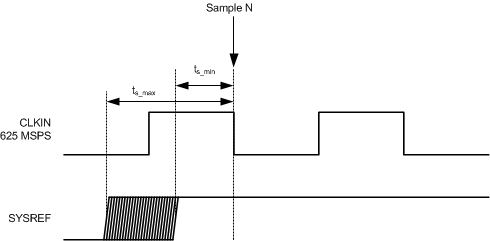Figure 1. SYSREF Timing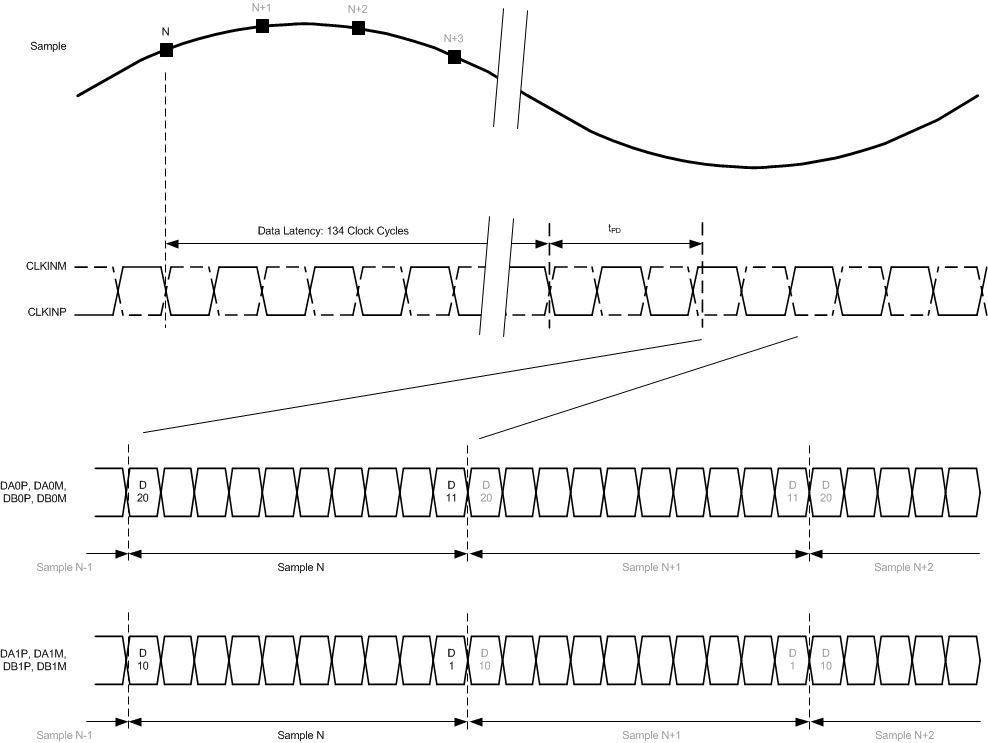Figure 2. Sample Timing Requirements

7.9 Typical Characteristics

typical values are at TA = 25°C, full temperature range is from TMIN = –40°C to TMAX = 85°C, ADC sampling rate = 625 MSPS, 50% clock duty cycle, AVDD3V = 3.0 V, AVDD = DVDD = 1.9 V, IOVDD = 1.15 V, –1-dBFS differential input, and 0-dB digital gain (unless otherwise noted)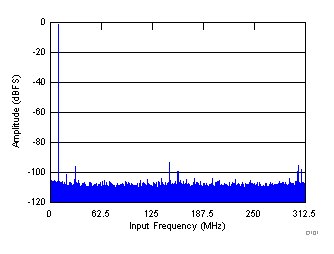SNR = 71.9 dBFS, SINAD = 71.86 dBFS, THD = 93 dBc, IL spur = 94 dBc, SFDR = 94 dBc, non HD2, HD3 spur = 94 dBc
Figure 3. FFT for 10-MHz Input Signal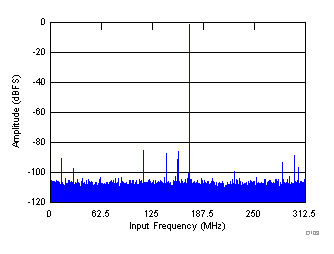SNR = 71 dBFS, SINAD = 70.9 dBFS, SFDR = 85 dBc, THD = 84 dBc, IL spur = 87 dBc, non HD2, HD3 spur = 93 dBc
Figure 5. FFT for 170-MHz Input Signal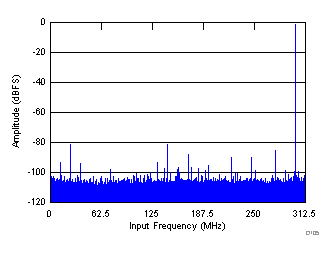SNR = 69.5 dBFS, SINAD = 69.1 dBFS, IL spur = 81 dBc, SFDR = 80 dBc, THD = 79 dBc, non HD2, HD3 spur = 90 dBc
Figure 7. FFT for 300-MHz Input Signal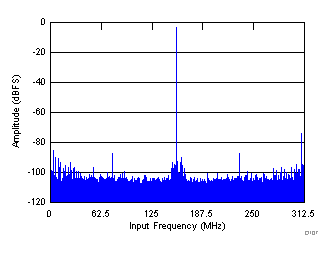Fundamental amplitude = –3 dBFS, SNR = 68.4 dBFS, SINAD = 66.1 dBFS, SFDR = 68 dBc, THD = 67 dBc, IL spur = 89 dBc, non HD2, HD3 spur = 85 dBc
Figure 9. FFT for 470-MHz Input Signal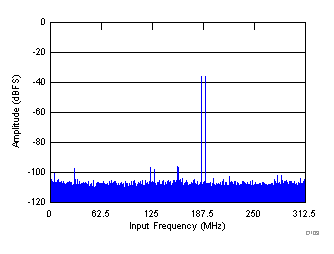fIN1 = 185 MHz, fIN2 = 190 MHz, each tone at –36 dBFS, IMD = 107 dBFS
Figure 11. FFT for Two-Tone Input Signal (–36 dBFS)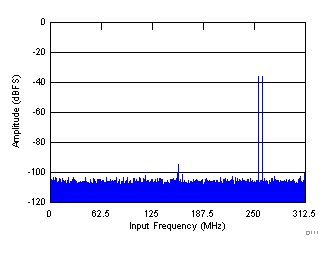fIN1 = 370 MHz, fIN2 = 365 MHz, each tone at –36 dBFS, IMD = 109 dBFS
Figure 13. FFT for Two-Tone Input Signal (–36 dBFS)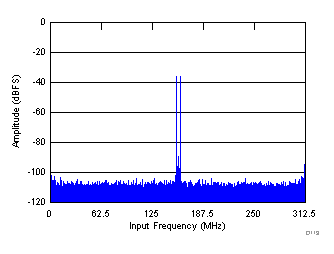fIN1 = 470 MHz, fIN2 = 465 MHz, each tone at –36 dBFS, IMD = 107 dBFS
Figure 15. FFT for Two-Tone Input Signal
(–36 dBFS)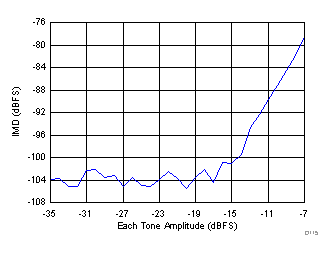fIN1 = 365 MHz, fIN2 = 370 MHz
Figure 17. Intermodulation Distortion vs Input Amplitude
(365 MHz and 370 MHz)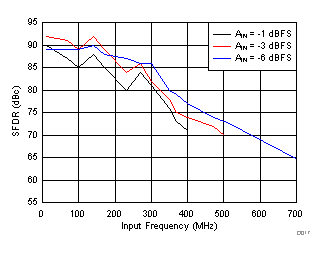Figure 19. Spurious-Free Dynamic Range vs
Input Frequency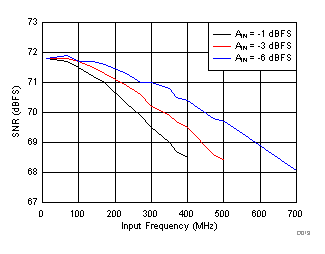Figure 21. Signal-to-Noise Ratio vs Input Frequency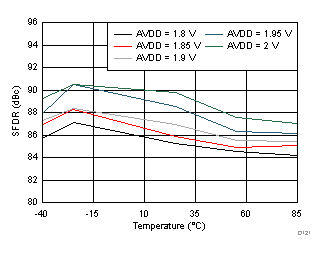fIN = 170 MHz
Figure 23. Spurious-Free Dynamic Range vs
AVDD Supply and Temperature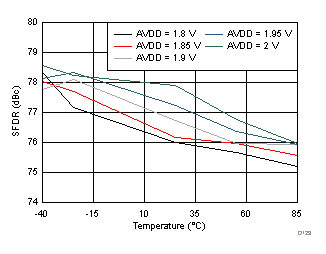fIN = 350 MHz
Figure 25. Spurious-Free Dynamic Range vs
AVDD Supply and Temperature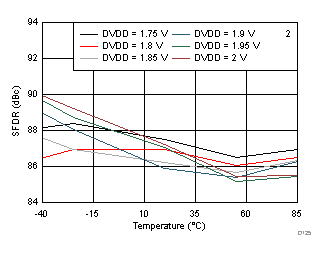fIN = 170 MHz
Figure 27. Spurious-Free Dynamic Range vs
DVDD Supply and Temperature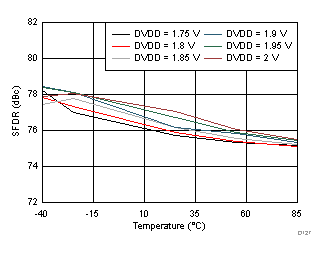fIN = 350 MHz
Figure 29. Spurious-Free Dynamic Range vs
DVDD Supply and Temperature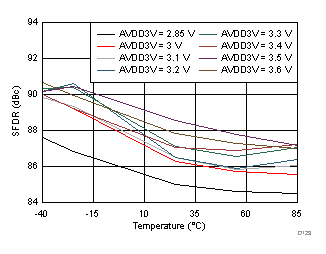fIN = 170 MHz
Figure 31. Spurious-Free Dynamic Range vs
AVDD3V Supply and Temperature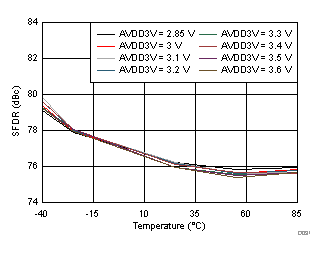fIN = 350 MHz
Figure 33. Spurious-Free Dynamic Range vs
AVDD3V Supply and Temperature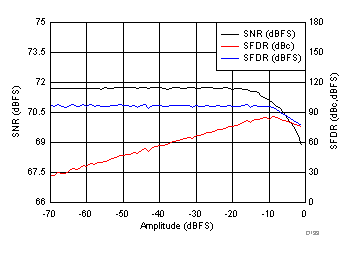fIN = 350 MHz
Figure 35. Performance vs Amplitude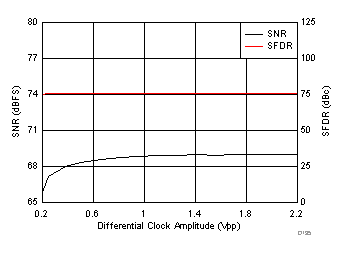fIN = 350 MHz
Figure 37. Performance vs Differential Clock AmplitudefIN = 350 MHz
Figure 39. Performance vs Input Clock Duty Cycle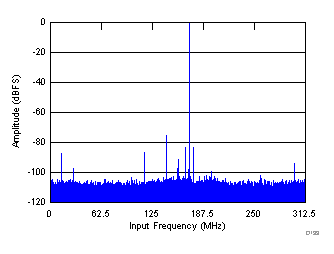fIN = 170 MHz, AIN = –1 dBFS, fPSRR = 5 MHz, APSRR= 50 mVPP, PSRR (AVDD supply) = 51 dB
Figure 41. Power-Supply Rejection Ratio FFT
for Test Signal on the AVDD Supply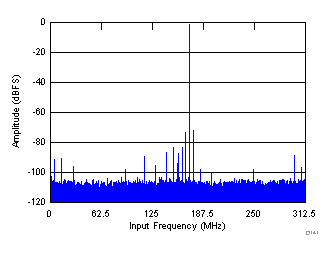fIN = 170 MHz, AIN = –1 dBFS, fCMRR = 5 MHz, ACMRR= 50 mVPP, CMRR = 40 dB
Figure 43. Common-Mode Rejection Ratio FFT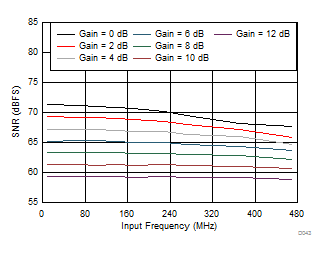NOTE: ADC output amplitude is –1 dBFS, input amplitude is scaled down by the amount of programmed digital gain.
Figure 45. Signal-to-Noise Ratio vs
Gain and Input Frequency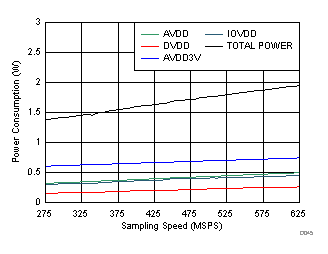Figure 47. Power Consumption vs Sampling SpeedSNR = 72 dBFS, SINAD = 71.8 dBFS, SFDR = 84 dBc, THD = 83 dBc, non HD2, HD3 spur = 98 dBc
Figure 49. FFT for 350-MHz Input Signal in
Decimate-by-2 Mode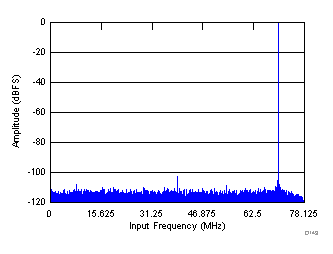SNR = 77.4 dBFS, SINAD = 77.3 dBFS, SFDR = 105 dBc, THD = 102 dBc, non HD2, HD3 spur = 105 dBc
Figure 51. FFT for 70-MHz Input Signal in
Decimate-by-4 Mode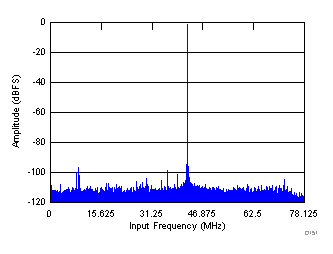SNR = 74.9 dBFS, SINAD = 74.8 dBFS, SFDR = 93 dBc, THD = 92 dBc, non HD2, HD3 spur = 93 dBc
Figure 53. FFT for 270-MHz Input Signal in
Decimate-by-4 Mode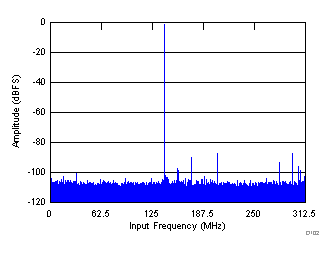SNR = 71.3 dBFS, SINAD = 71.1 dBFS, SFDR = 86 dBc, THD = 85 dBc, IL spur = 87 dBc, non HD2, HD3 spur = 95 dBc
Figure 4. FFT for 140-MHz Input Signal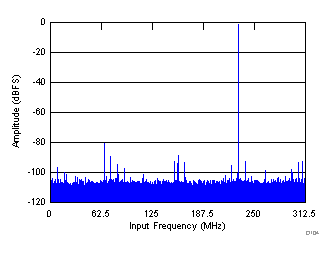SNR = 70.4 dBFS, SINAD = 69.9 dBFS, IL spur = 89 dBc, SFDR = 80 dBc, THD = 79 dBc, non HD2, HD3 spur = 91 dBc
Figure 6. FFT for 230-MHz Input SignalSNR = 68.8 dBFS, SINAD = 67.3 dBFS, SFDR = 73 dBc, THD = 72 dBc, IL spur = 81 dBc, non HD2, HD3 spur = 89 dBc
Figure 8. FFT for 370-MHz Input Signal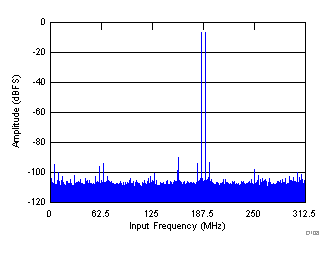fIN1 = 185 MHz, fIN2 = 190 MHz, each tone at –7 dBFS, IMD = 89 dBFS
Figure 10. FFT for Two-Tone Input Signal (–7 dBFS)fIN1 = 370 MHz, fIN2 = 365 MHz, each tone at –7 dBFS, IMD = 78 dBFS
Figure 12. FFT for Two-Tone Input Signal (–7 dBFS)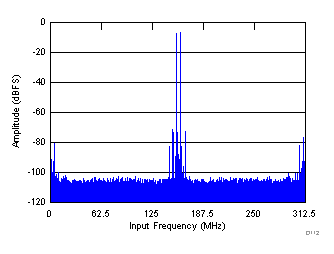fIN1 = 470 MHz, fIN2 = 465 MHz, each tone at –7 dBFS, IMD = 71 dBFS
Figure 14. FFT for Two-Tone Input Signal (–7 dBFS)fIN1 = 185 MHz, fIN2 = 190 MHz
Figure 16. Intermodulation Distortion vs Input Amplitude
(185 MHz and 190 MHz)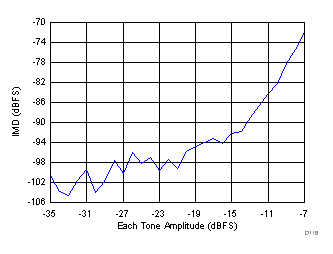fIN1 = 465 MHz, fIN2 = 470 MHz
Figure 18. Intermodulation Distortion vs Input Amplitude
(465 MHz and 470 MHz)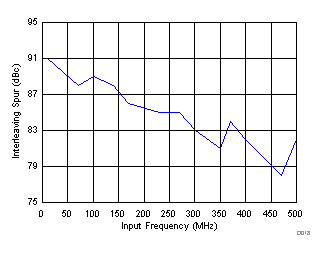Figure 20. Interleaving Spur vs Input Frequency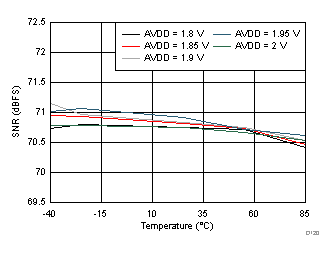fIN = 170 MHz
Figure 22. Signal-to-Noise Ratio vs
AVDD Supply and Temperature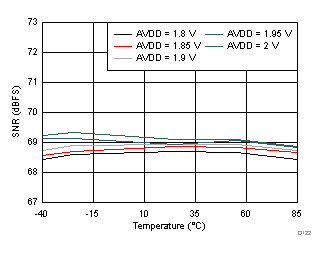fIN = 350 MHz
Figure 24. Signal-to-Noise Ratio vs
AVDD Supply and Temperature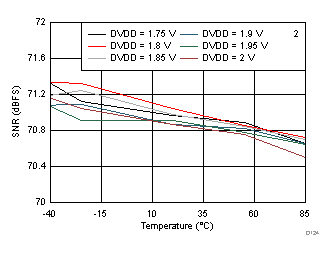fIN = 170 MHz
Figure 26. Signal-to-Noise Ratio vs
DVDD Supply and Temperature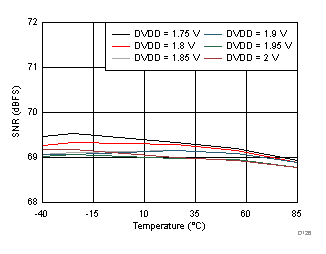fIN = 350 MHz
Figure 28. Signal-to-Noise Ratio vs
DVDD Supply and Temperature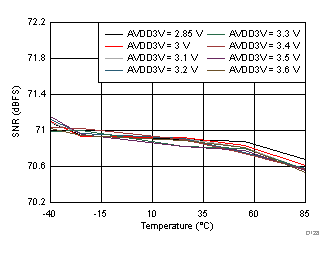fIN = 170 MHz
Figure 30. Signal-to-Noise Ratio vs
AVDD3V Supply and Temperature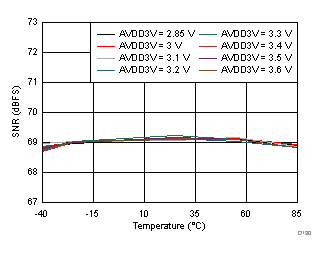fIN = 350 MHz
Figure 32. Signal-to-Noise Ratio vs
AVDD3V Supply and Temperature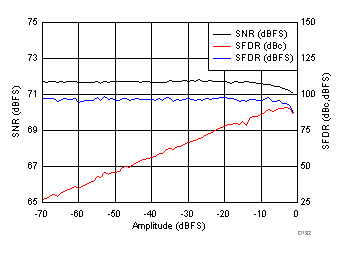fIN = 170 MHz
Figure 34. Performance vs AmplitudefIN = 170 MHz
Figure 36. Performance vs Differential Clock Amplitude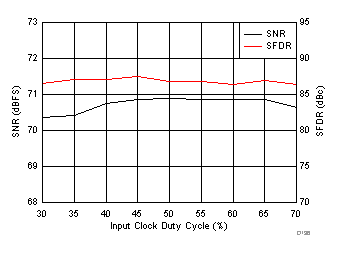fIN = 170 MHz
Figure 38. Performance vs Input Clock Duty Cycle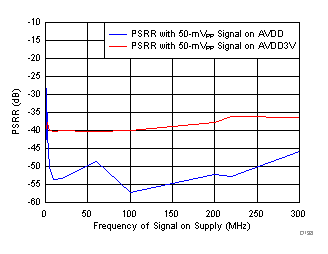Figure 40. Power-Supply Rejection Ratio vs
Test Signal on AVDD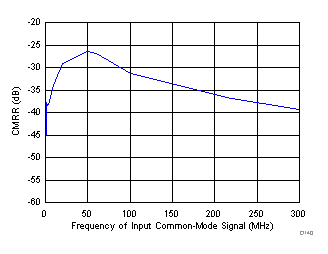Figure 42. Common-Mode Rejection Ratio vs
Common-Mode Signal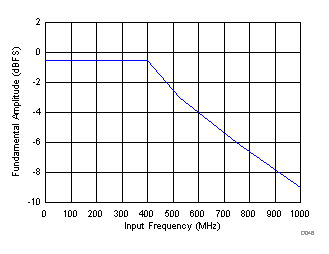Figure 44. Maximum-Supported Amplitude vs
Input Frequency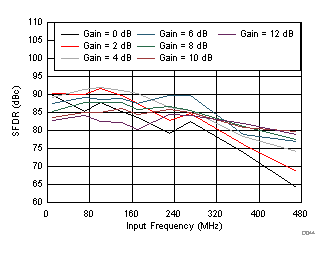NOTE: ADC output amplitude is –1 dBFS, input amplitude is scaled down by the amount of programmed digital gain.
Figure 46. Spurious-Free Dynamic Range vs
Gain and Input Frequency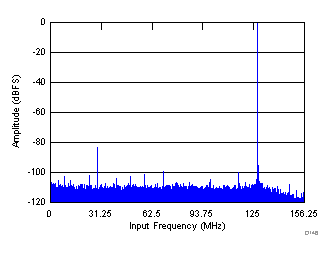SNR = 74.1 dBFS, SINAD = 74.09 dBFS, SFDR = 98 dBc, THD = 93 dBc, non HD2, HD3 spur = 99 dBc
Figure 48. FFT for 185-MHz Input Signal in
Decimate-by-2 Mode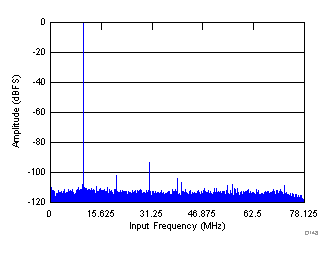SNR = 77.6 dBFS, SINAD = 77.5 dBFS, SFDR = 93 dBc, THD = 92 dBc, non HD2, HD3 spur = 106 dBc
Figure 50. FFT for 10-MHz Input Signal in
Decimate-by-4 Mode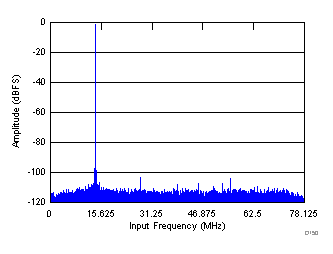SNR = 76.7 dBFS, SINAD = 76.6 dBFS, SFDR = 96 dBc, THD = 98 dBc, non HD2, HD3 spur = 96 dBc
Figure 52. FFT for 170-MHz Input Signal in
Decimate-by-4 Mode

7.10 Typical Characteristics: Contour

typical values are at TA = 25°C, full temperature range is from TMIN = –40°C to TMAX = 85°C, ADC sampling rate = 625 MSPS, 50% clock duty cycle, AVDD3V = 3.0 V, AVDD = DVDD = 1.9 V, IOVDD = 1.15 V, –1-dBFS differential input, and 0-dB digital gain (unless otherwise noted)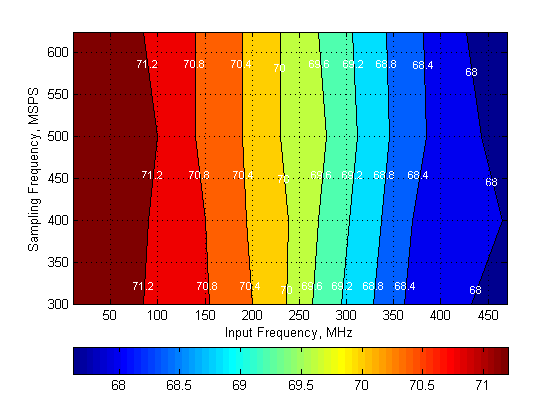Figure 54. Signal-to-Noise-Ratio with 0-dB Digital Gain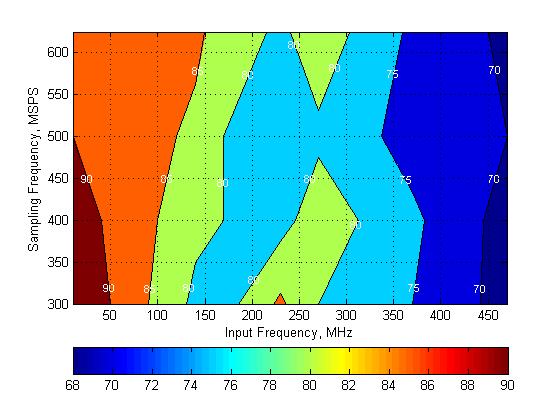Figure 56. Spurious-Free-Dynamic-Range with
0-dB Digital Gain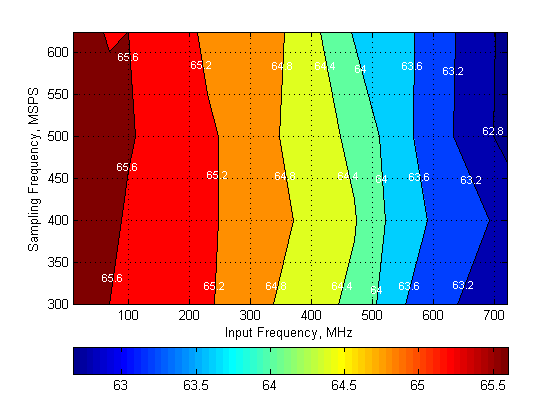Figure 55. Signal-to-Noise-Ratio with
6-dB Digital Gain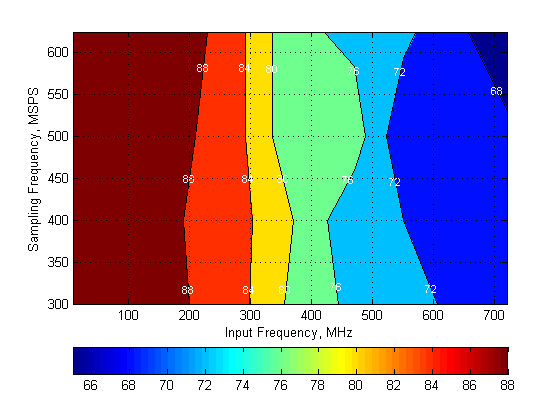Figure 57. Spurious-Free-Dynamic-Range with
6-dB Digital Gain